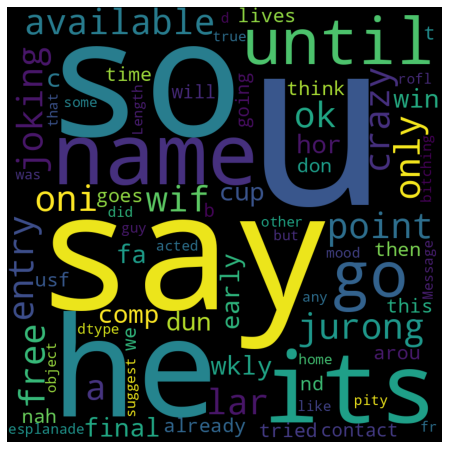# Exploring NLP with a SPAM filter classifier using SVM¶

We are going to build a machine learneing model that can identify whether or not an email is spam, so this is a binary classification problem. I am going to use the Python library Scikit-Learn to explore tokenization, vectorization, and statistical classification algorithms. Let's begin by importing the necessary dependencies such as CountVectorizer, SVM, and a couple of metrics.

Importing dependencies

In :
import pandas as pd
import regex as re
import matplotlib.pyplot as plt
from wordcloud import WordCloud

from sklearn.feature_extraction.text import CountVectorizer
from sklearn.model_selection import train_test_split
from sklearn import model_selection, svm
from sklearn.metrics import classification_report, accuracy_score


In :
df = pd.read_csv("https://raw.githubusercontent.com/4GeeksAcademy/machine-learning-content/master/assets/spam.csv")

In :
#Looking at the first rows


Out:
Category Message
0 ham Go until jurong point, crazy.. Available only ...
1 ham Ok lar... Joking wif u oni...
2 spam Free entry in 2 a wkly comp to win FA Cup fina...
3 ham U dun say so early hor... U c already then say...
4 ham Nah I don't think he goes to usf, he lives aro...

In this example, we have decided to convert our target variable into numbers right away. Spam will become 1 and ham will become 0.

In :
df['Category'] = df['Category'].apply(lambda x: 1 if x == 'spam' else 0)

In :
df.head()

Out:
Category Message
0 0 Go until jurong point, crazy.. Available only ...
1 0 Ok lar... Joking wif u oni...
2 1 Free entry in 2 a wkly comp to win FA Cup fina...
3 0 U dun say so early hor... U c already then say...
4 0 Nah I don't think he goes to usf, he lives aro...

Cleaning Process

We already learned how to clean the data, so we will start by counting spam and not spam emails, and also eliminating duplicates.

In :
# EDA: Establish some baseline counts and eliminate duplicate rows.

print("spam count: " +str(len(df.loc[df.Category==1])))
print("not spam count: " +str(len(df.loc[df.Category==0])))
print(df.shape)
df['Category'] = df['Category'].astype(int)

spam count: 747
not spam count: 4825
(5572, 2)

In :
df = df.drop_duplicates()
df = df.reset_index(inplace = False)[['Message','Category']]
df.shape

Out:
(5157, 2)

Observations: We have dropped 415 duplicated rows.

Using regular expressions let's convert all words to lower case, clean the data from punctuation marks and special characters.

In :
clean_desc = []

for w in range(len(df.Message)):
desc = df['Message'][w].lower()

#remove punctuation
desc = re.sub('[^a-zA-Z]', ' ', desc)

#remove tags
desc=re.sub("&lt;/?.*?&gt;"," &lt;&gt; ",desc)

#remove digits and special chars
desc=re.sub("(\\d|\\W)+"," ",desc)

clean_desc.append(desc)

#assign the cleaned descriptions to the data frame
df['Message'] = clean_desc


Out:
Message Category
0 go until jurong point crazy available only in ... 0
1 ok lar joking wif u oni 0
2 free entry in a wkly comp to win fa cup final ... 1

Let's build a stop words list from scratch and then visualize our wordcloud.

In :
# Building a stop words list from scratch

stop_words = ['is','you','your','and', 'the', 'to', 'from', 'or', 'I', 'for', 'do', 'get', 'not', 'here', 'in', 'im', 'have', 'on',
're', 'new', 'subject']

In :
wordcloud = WordCloud(width = 800, height = 800, background_color = 'black', stopwords = stop_words, max_words = 1000
, min_font_size = 20).generate(str(df['Message']))
#plot the word cloud
fig = plt.figure(figsize = (8,8), facecolor = None)
plt.imshow(wordcloud)
plt.axis('off')
plt.show()Now that the Messages have been cleaned, to prevent the word cloud from outputting sentences, the messages go through a process called tokenization. It is the process of breaking down a sentence into the individual words. The individual words are called tokens.

Vectorizing

Using SciKit-Learn’s CountVectorizer(), it is easy to transform the body of text into a sparse matrix of numbers that the computer can pass to machine learning algorithms. We are going to create the sparse matrix, then split the data using sk-learn train_test_split().

In :
#Create sparse matrix

message_vectorizer = CountVectorizer().fit_transform(df['Message'])

# Split the data

X_train, X_test, y_train, y_test = train_test_split(message_vectorizer, df['Category'], test_size = 0.45, random_state = 42, shuffle = True)


The classifier algorithm

In this example we will practice using Support Vector Machine but feel free to try addtional classifiers.

In :
classifier = svm.SVC(C=1.0, kernel='linear', degree=3, gamma='auto')


Now let's generate some predictions

In :
classifier.fit(X_train, y_train)
predictions = classifier.predict(X_test)
print(classification_report(y_test, predictions))

              precision    recall  f1-score   support

0       0.98      1.00      0.99      2024
1       0.96      0.89      0.92       297

accuracy                           0.98      2321
macro avg       0.97      0.94      0.96      2321
weighted avg       0.98      0.98      0.98      2321


In :
# Use accuracy_score function to get the accuracy

print("SVM Accuracy Score -> ",accuracy_score(predictions, y_test)*100)

SVM Accuracy Score ->  98.10426540284361


Our model achieved 98% accuracy!